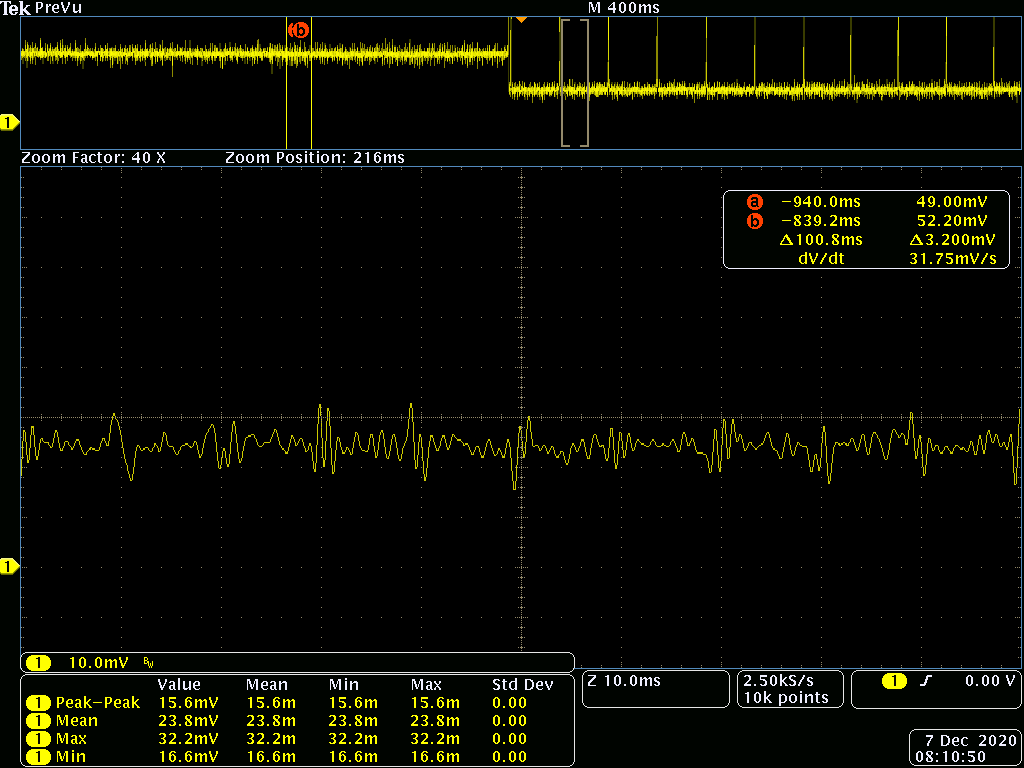If you have a related question, please click the "Ask a related question" button in the top right corner. The newly created question will be automatically linked to this question.

# INA216: INA216 Calculating current consumption

Part Number: INA216

I am using INA216EVM and the amplifier with a gain of 200. The current shunt resistor is 0.04 ohms.

I am measuring the output on the scope. However, I am don't know how to calculate (formula) the current consumption from mV.

On the power supply, I notice about 1mA but am looking for more precise value in uA. Can you advise the formula to calculate.• The supply voltage is 3V.

• Hi,

30µA maximum for the INA216 itself, as mentioned in the datasheet, plus the current into the load connected to the output of INA216. As an example: 3V into 10k gives additional 300µA :-)

Kai

• I am looking to convert from mV (from Scope) to mA. For example, the mean voltage on scope is 23.8 mV. How do I convert this to mA?

• Hello RBM,

Thanks for considering to use Texas Instruments.  To calculate the equivalent current, you take the INA216 output and divide by the gain and then divide by the shunt value.  So 0.0238V/(200V/V)/(0.04Ω)=.002975A=2.975mA.  Since your measured current is so low (at least for this device), you will have see some significant error from the device.  Two related specs that dominate at low current levels are voltage offset and input bias current.  Assuming your common mode is similar to the conditions in the datasheet electrical characteristics table for voltage offset, your typical error would be (30E-6V)/((0.0238V)/(200V/V))*100%= 25%.  Worst case would be even greater.  You can do an initial no load condition measurement to help you account for this issue.